• 条形图 比较条形图条形图、简单条形图 #Develop By #Ahsan Zahid Chowdhury # #+880-1534-302690JavaScript
• ## python 画条形图（柱状图）

万次阅读 多人点赞 2019-08-24 00:06:35
竖放条形图bar(x, height, [width], **kwargs)2. 画横放条形图bar(x, width, [height], **kwargs)3. 并列条形图 条形图（bar chart），也称为柱状图，是一种以长方形的长度为变量的统计图表，长方形的长度与它所...


文章目录
1. 竖放条形图bar(x, height, [width], **kwargs)

2. 横放条形图bar(x, width, [height], **kwargs)

3. 并列条形图

条形图（bar chart），也称为柱状图，是一种以长方形的长度为变量的统计图表，长方形的长度与它所对应的变量数值呈一定比例。

1. 竖放条形图
画条形图要用到 pyplot 中的 bar 函数，该函数的基本语法为：

bar(x, height, [width], **kwargs)

x数组，每个条形的横坐标height个数或一个数组，条形的高度[width]可选参数，一个数或一个数组，条形的宽度，默认为 0.8**kwargs不定长的关键字参数，用字典形式设置条形图的其他属性
**kwargs 中常设置的参数包括图形标签 label，颜色标签 color，不透明度 alpha 等。
假设某项针对男女大学生购买饮用水爱好的调查结果如下表：
男女碳酸饮料69绿茶74矿泉水64其他26果汁15总计2228
画出男生饮用水情况的直方图，代码如下：
import matplotlib.pyplot as plt

# 这两行代码解决 plt 中文显示的问题
plt.rcParams['font.sans-serif'] = ['SimHei']
plt.rcParams['axes.unicode_minus'] = False

waters = ('碳酸饮料', '绿茶', '矿泉水', '果汁', '其他')
buy_number = [6, 7, 6, 1, 2]

plt.title('男性购买饮用水情况的调查结果')

plt.show()

图形：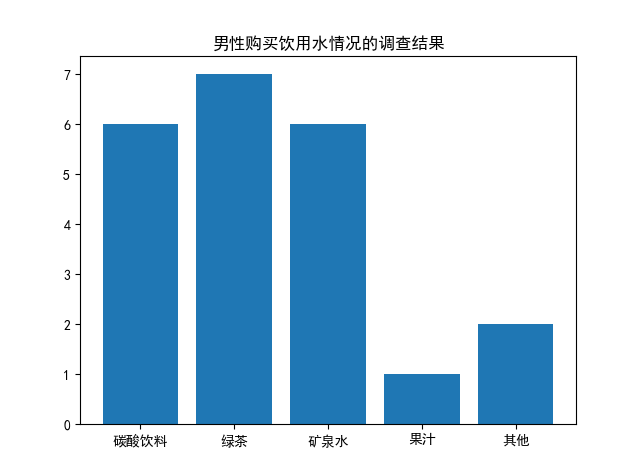2. 横放条形图
若要生成横的条形图，则可以使用 barh 函数，其语法与 bar 函数非常类似。

bar(x, width, [height], **kwargs)

y数组，每个条形的纵坐标width一个数或一个数组，条形的宽度[height]可选参数，一个数或一个数组，条形的高度，默认为 0.8**kwargs不定长的关键字参数，用字典形式设置条形图的其他属性
代码：
import matplotlib.pyplot as plt

# 这两行代码解决 plt 中文显示的问题
plt.rcParams['font.sans-serif'] = ['SimHei']
plt.rcParams['axes.unicode_minus'] = False

waters = ('碳酸饮料', '绿茶', '矿泉水', '果汁', '其他')
buy_number = [6, 7, 6, 1, 2]

plt.title('男性购买饮用水情况的调查结果')

plt.show()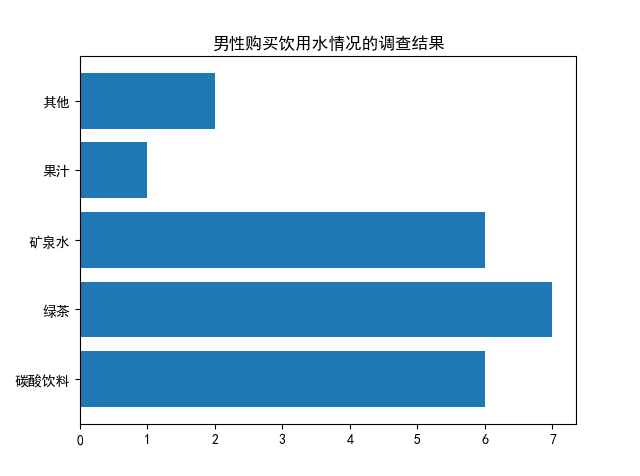3. 并列条形图
若要将男生与女生的调查情况画出两个条形图一块显示，则可以使用 bar 或 barh 函数两次，并调整 bar 或 barh 函数的条形图位置坐标以及相应刻度，使得两组条形图能够并排显示。
import matplotlib.pyplot as plt
import numpy as np

# 这两行代码解决 plt 中文显示的问题
plt.rcParams['font.sans-serif'] = ['SimHei']
plt.rcParams['axes.unicode_minus'] = False

# 输入统计数据
waters = ('碳酸饮料', '绿茶', '矿泉水', '果汁', '其他')
buy_number_male = [6, 7, 6, 1, 2]
buy_number_female = [9, 4, 4, 5, 6]

bar_width = 0.3  # 条形宽度
index_male = np.arange(len(waters))  # 男生条形图的横坐标
index_female = index_male + bar_width  # 女生条形图的横坐标

# 使用两次 bar 函数画出两组条形图

plt.legend()  # 显示图例
plt.xticks(index_male + bar_width/2, waters)  # 让横坐标轴刻度显示 waters 里的饮用水， index_male + bar_width/2 为横坐标轴刻度的位置
plt.ylabel('购买量')  # 纵坐标轴标题
plt.title('购买饮用水情况的调查结果')  # 图形标题

plt.show()

显示：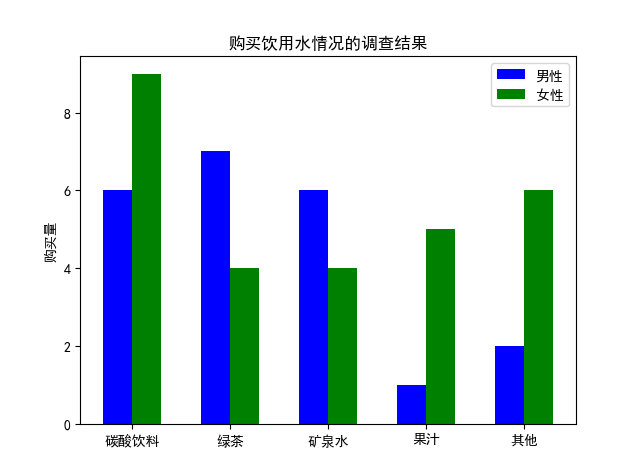展开全文python 柱状图 bar
•JavaScript
• Excel柱形图条形图模板-销量地区分布图堆积条形图
• Excel柱形图条形图模板-截断条形图圆柱图食品销量分析
• Excel柱形图条形图模板-经济学人条形图圆柱分析图
• Excel柱形图条形图模板-绿色清新条形图设计Excel图表
• matlab开发-BarPatchHighly可定制条形图条形图。创建并自定义带有误差线的条形图。支持分组数据。
• Excel柱形图条形图模板-自动突出后三名条形图Excel图表
• Excel柱形图条形图模板-图表模板-月份对比条形图Excel图表
• 一、Bar 条形图、 1、bar 函数、 2、矩阵数据表示、 3、bar 函数代码示例、 二、Bar 条形图样式、 1、bar 函数样式、 2、堆叠条形图示例、 三、水平条形图、 1、barh 函数、 2、代码示例、


文章目录
一、Bar 条形图1、bar 函数2、矩阵数据表示3、bar 函数代码示例
二、Bar 条形图样式1、bar 函数样式2、堆叠条形图示例
三、水平条形图1、barh 函数2、代码示例

一、Bar 条形图

1、bar 函数

bar 函数参考文档 : https://ww2.mathworks.cn/help/matlab/ref/bar.html

2、矩阵数据表示

x

x

值是一个矩阵 :

x

=

[

1

2

5

4

8

]

x = \begin{bmatrix} 1 & 2 & 5 & 4 & 8 \end{bmatrix}

代码表示例 :
% 条形图的数值列表
x = [1 , 2 , 5 , 4 , 8]


y

y

是

x

x

与另一个矩阵组成的新矩阵 :

y

=

[

1

2

5

4

8

1

2

3

4

5

]

y = \begin{bmatrix} 1 & 2 & 5 & 4 & 8 \\\\ 1 & 2 & 3 & 4 & 5 \end{bmatrix}

代码示例 :
% 数值列表 , 组成一个矩阵
y = [x; 1:5];


3、bar 函数代码示例

bar 函数代码示例 :
% 条形图的数值列表
x = [1, 2, 5, 4, 8];

% 数值列表 , 组成一个矩阵
y = [x; 1:5];

% 绘制第一张图像
subplot(3, 1, 1);

% 绘制条形图 , x 中每个元素对应一个条形
bar(x);

% 绘制第二张图像
subplot(3, 1, 2);

% 绘制条形图 , y 中每个元素对应一个条形
% y 是个矩阵 , 有 2 x 5 = 10 个元素
bar(y);

% 绘制第三张图像
subplot(3, 1, 3);

% 绘制立体 3D 条形图 , y 中每个元素对应一个条形
% y 是个矩阵 , 有 2 x 5 = 10 个元素
bar3(y);


代码示例 :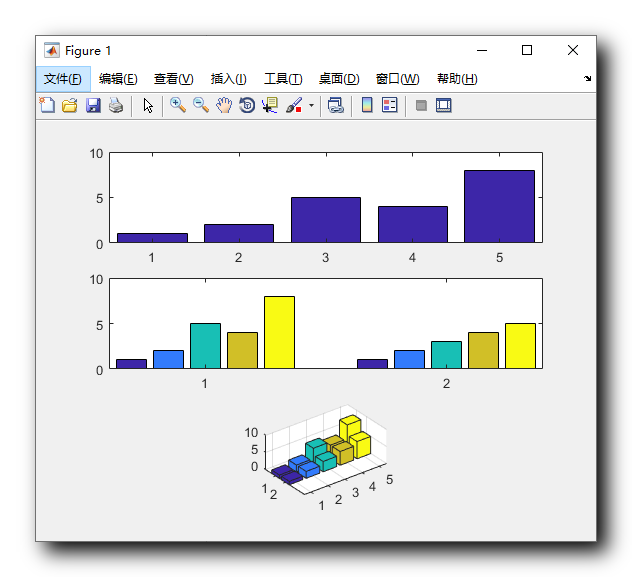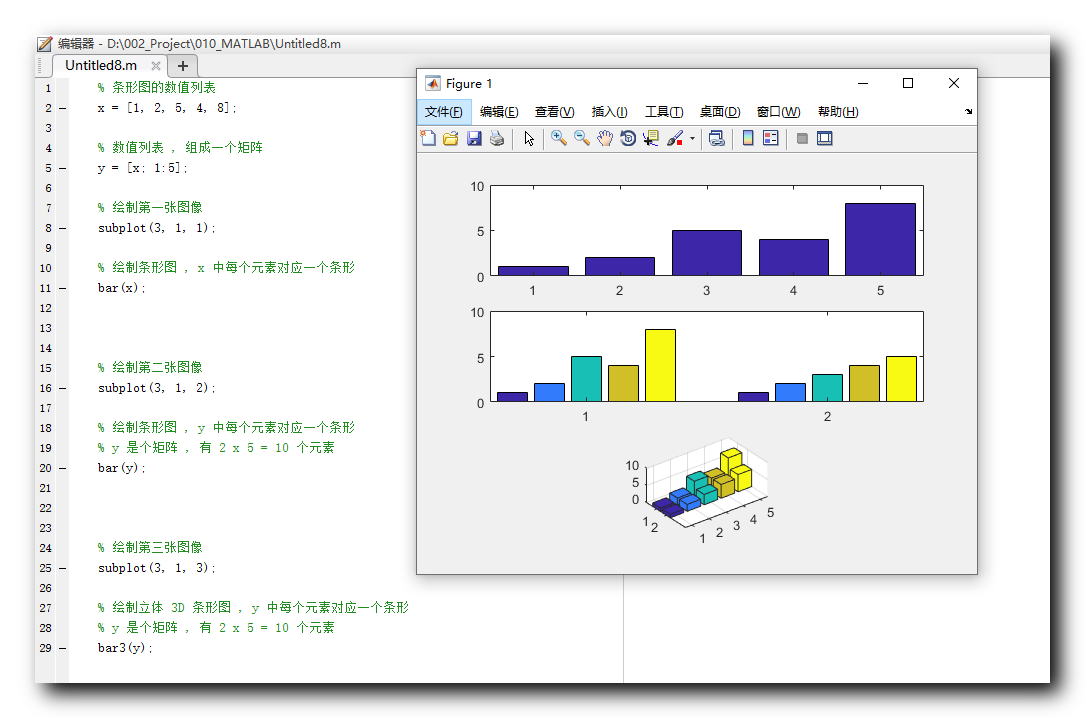二、Bar 条形图样式

bar 函数参考文档 : https://ww2.mathworks.cn/help/matlab/ref/bar.html

1、bar 函数样式

在 bar 函数的数据后面 , 可以使用字符串指定一个条形图样式 ,
条形图的四种样式如下 :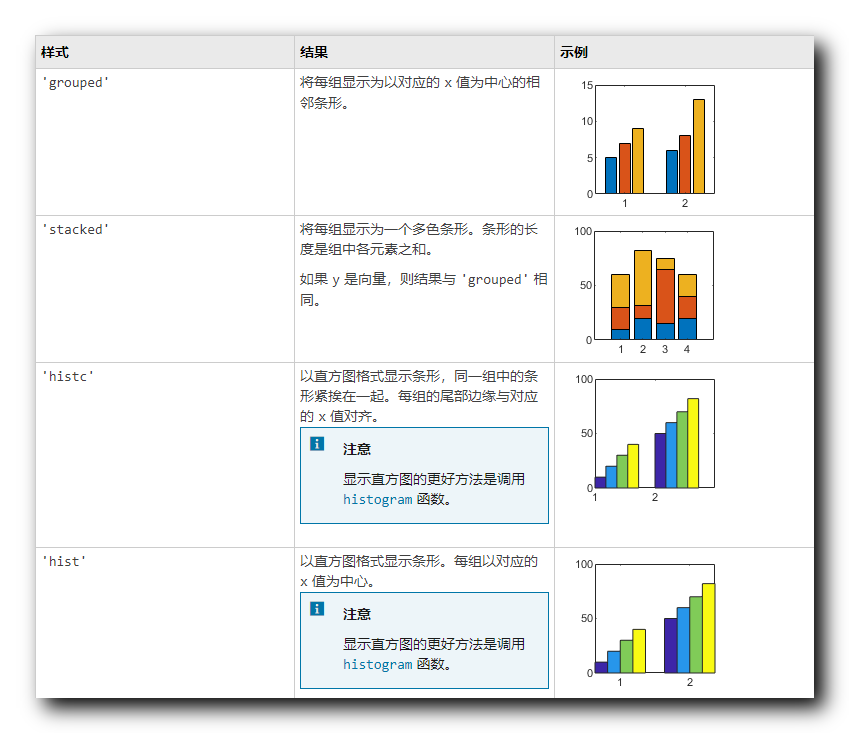2、堆叠条形图示例

% 条形图的数值列表
x = [1, 2, 5, 4, 8];

% 数值列表 , 组成一个矩阵
y = [x; 1:5];

% 绘制第一张图像
subplot(2, 1, 1);

% 绘制条形图 , y 中每个元素对应一个条形
% y 是个矩阵 , 有 2 x 5 = 10 个元素
bar(y);

% 绘制第二张图像
subplot(2, 1, 2);

% 绘制条形图 , y 中每个元素对应一个条形
% y 是个矩阵 , 有 2 x 5 = 10 个元素
bar(y, 'stacked');


绘图效果 :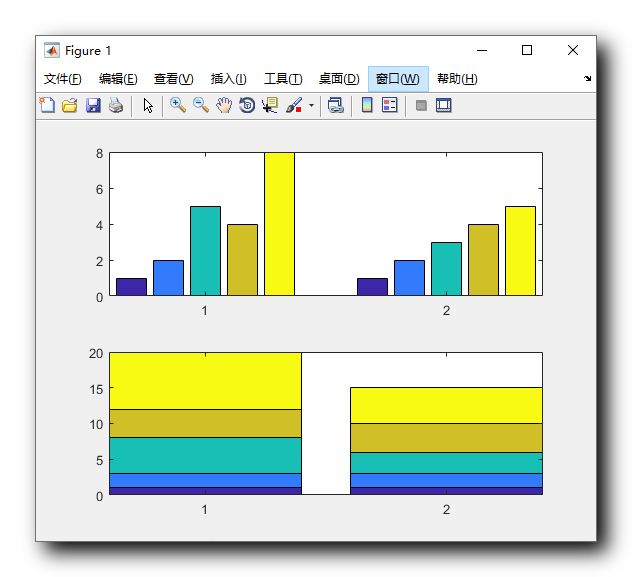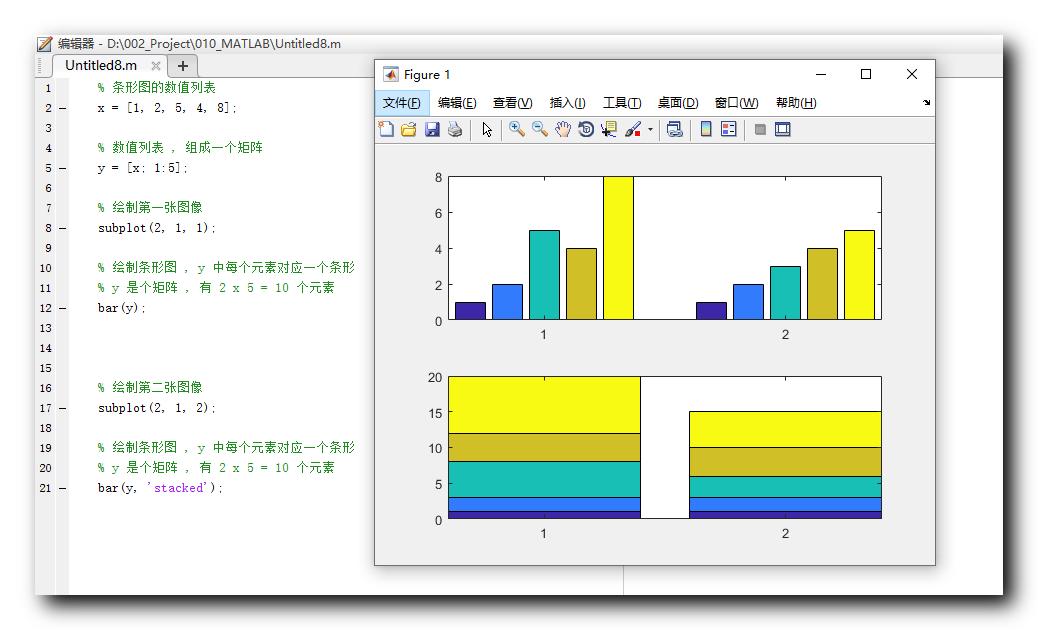三、水平条形图

barh 函数参考文档 : https://ww2.mathworks.cn/help/matlab/ref/barh.html

1、barh 函数

与 bar 用法类似 , 使用 barh 函数绘制的条形图是水平条形图 ;

2、代码示例

代码示例 :
% 条形图的数值列表
x = [1, 2, 5, 4, 8];

% 数值列表 , 组成一个矩阵
y = [x; 1:5];

% 绘制第一张图像
subplot(2, 1, 1);

% 绘制条形图 , y 中每个元素对应一个条形
% y 是个矩阵 , 有 2 x 5 = 10 个元素
barh(y);

% 绘制第二张图像
subplot(2, 1, 2);

% 绘制条形图 , y 中每个元素对应一个条形
% y 是个矩阵 , 有 2 x 5 = 10 个元素
barh(y, 'stacked');


绘图结果 :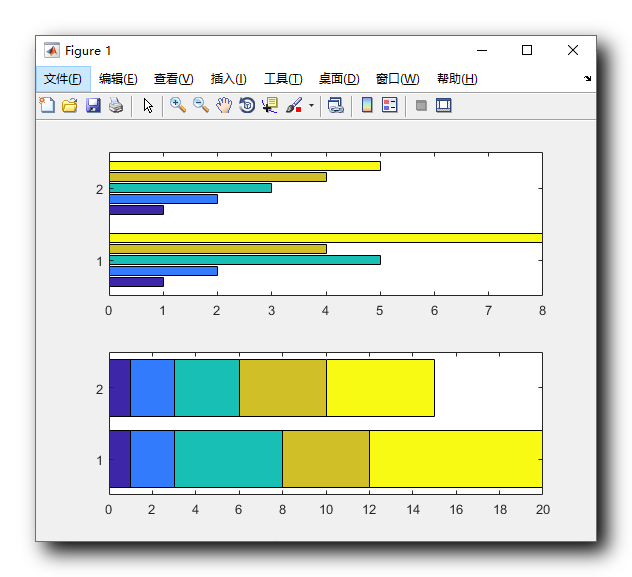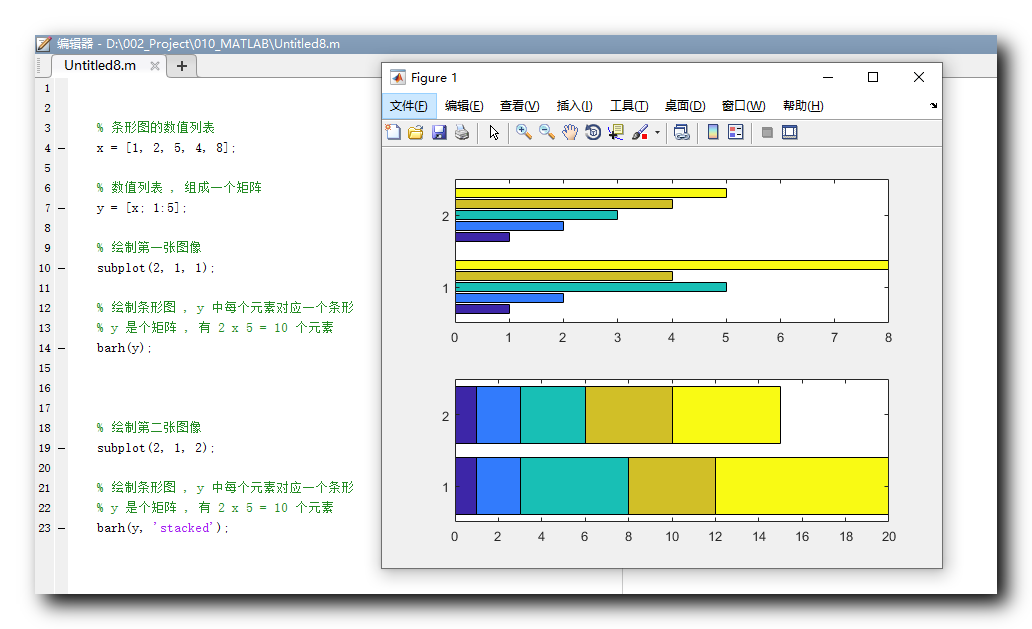展开全文• labview条形图，自己用着反正可以，应该是个老资源了吧
• android 条形图 1.展示条形图或折线图 2.绘制启动动画
• matplotlib绘制直方图、条形图和饼图 matplotlib是一个非常强大的Python第三方绘图包，可以绘制非常多非常漂亮的图形。matplotlib.pyplot提供了一个类Matlab的函数式编程环境。通常的绘图任务，都可以通过...
matplotlib绘制直方图、条形图和饼图
matplotlib是一个非常强大的Python第三方绘图包，可以绘制非常多非常漂亮的图形。matplotlib.pyplot提供了一个类Matlab的函数式编程环境。通常的绘图任务，都可以通过matplotlib.pyplot完成，下面将记录下绘制直方图和饼图的过程。
1 绘制直方图
首先需要区分清楚概念：直方图和条形图。
条形图：条形图用长条形表示每一个类别，长条形的长度表示类别的频数，宽度表示表示类别。直方图：直方图是一种统计报告图，形式上也是一个个的长条形，但是直方图用长条形的面积表示频数，所以长条形的高度表示

频数组距
$\frac{频数}{组距}$，宽度表示组距，其长度和宽度均有意义。当宽度相同时，一般就用长条形长度表示频数。
直方图一般用来描述等距数据，柱状图一般用来描述名称（类别）数据或顺序数据。直观上，直方图各个长条形是衔接在一起的，表示数据间的数学关系；条形图各长条形之间留有空隙，区分不同的类。
区别频数分布直方图条形图横轴上的数据连续的，是一个范围孤立的，代表一个类别长条形之间没有空隙有空隙频数的表示一般用长条形面积表示；当宽度相同时，用长度表示长条形的长度
1.1直方图
import matplotlib.pyplot as plt
import numpy as np
import matplotlib

# 设置matplotlib正常显示中文和负号
matplotlib.rcParams['font.sans-serif']=['SimHei']   # 用黑体显示中文
matplotlib.rcParams['axes.unicode_minus']=False     # 正常显示负号
# 随机生成（10000,）服从正态分布的数据
data = np.random.randn(10000)
"""
绘制直方图
data:必选参数，绘图数据
bins:直方图的长条形数目，可选项，默认为10
normed:是否将得到的直方图向量归一化，可选项，默认为0，代表不归一化，显示频数。normed=1，表示归一化，显示频率。
facecolor:长条形的颜色
edgecolor:长条形边框的颜色
alpha:透明度
"""
plt.hist(data, bins=40, normed=0, facecolor="blue", edgecolor="black", alpha=0.7)
# 显示横轴标签
plt.xlabel("区间")
# 显示纵轴标签
plt.ylabel("频数/频率")
# 显示图标题
plt.title("频数/频率分布直方图")
plt.show()
绘制的直方图效果如下：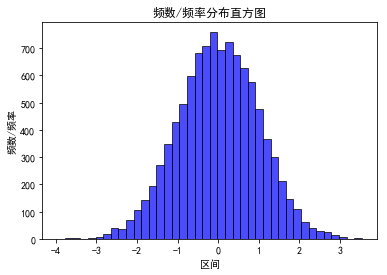1.2条形图
import matplotlib.pyplot as plt
import matplotlib
# 设置中文字体和负号正常显示
matplotlib.rcParams['font.sans-serif'] = ['SimHei']
matplotlib.rcParams['axes.unicode_minus'] = False

label_list = ['2014', '2015', '2016', '2017']    # 横坐标刻度显示值
num_list1 = [20, 30, 15, 35]      # 纵坐标值1
num_list2 = [15, 30, 40, 20]      # 纵坐标值2
x = range(len(num_list1))
"""
绘制条形图
left:长条形中点横坐标
height:长条形高度
width:长条形宽度，默认值0.8
label:为后面设置legend准备
"""
rects1 = plt.bar(left=x, height=num_list1, width=0.4, alpha=0.8, color='red', label="一部门")
rects2 = plt.bar(left=[i + 0.4 for i in x], height=num_list2, width=0.4, color='green', label="二部门")
plt.ylim(0, 50)     # y轴取值范围
plt.ylabel("数量")
"""
设置x轴刻度显示值
参数一：中点坐标
参数二：显示值
"""
plt.xticks([index + 0.2 for index in x], label_list)
plt.xlabel("年份")
plt.title("某某公司")
plt.legend()     # 设置题注
# 编辑文本
for rect in rects1:
height = rect.get_height()
plt.text(rect.get_x() + rect.get_width() / 2, height+1, str(height), ha="center", va="bottom")
for rect in rects2:
height = rect.get_height()
plt.text(rect.get_x() + rect.get_width() / 2, height+1, str(height), ha="center", va="bottom")
plt.show()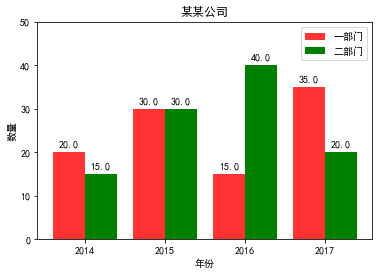1.3 水平条形图
import matplotlib.pyplot as plt
import matplotlib

matplotlib.rcParams['font.sans-serif'] = ['SimHei']
matplotlib.rcParams['axes.unicode_minus'] = False

price = [39.5, 39.9, 45.4, 38.9, 33.34]
"""
绘制水平条形图方法barh
参数一：y轴
参数二：x轴
"""
plt.barh(range(5), price, height=0.7, color='steelblue', alpha=0.8)      # 从下往上画
plt.yticks(range(5), ['亚马逊', '当当网', '中国图书网', '京东', '天猫'])
plt.xlim(30,47)
plt.xlabel("价格")
plt.title("不同平台图书价格")
for x, y in enumerate(price):
plt.text(y + 0.2, x - 0.1, '%s' % y)
plt.show()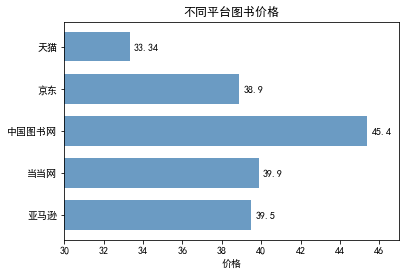1.4 堆叠条形图
import matplotlib.pyplot as plt
import matplotlib

matplotlib.rcParams['font.sans-serif'] = ['SimHei']
matplotlib.rcParams['axes.unicode_minus'] = False

label_list = ['2014', '2015', '2016', '2017']
num_list1 = [20, 30, 15, 35]
num_list2 = [15, 30, 40, 20]
x = range(len(num_list1))
rects1 = plt.bar(left=x, height=num_list1, width=0.45, alpha=0.8, color='red', label="一部门")
rects2 = plt.bar(left=x, height=num_list2, width=0.45, color='green', label="二部门", bottom=num_list1)
plt.ylim(0, 80)
plt.ylabel("数量")
plt.xticks(x, label_list)
plt.xlabel("年份")
plt.title("某某公司")
plt.legend()
plt.show()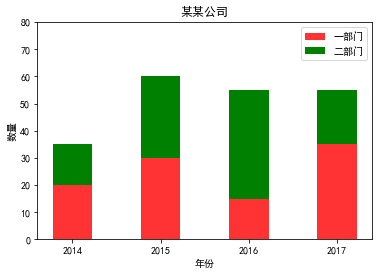饼图
import matplotlib.pyplot as plt
import matplotlib

matplotlib.rcParams['font.sans-serif'] = ['SimHei']
matplotlib.rcParams['axes.unicode_minus'] = False

label_list = ["第一部分", "第二部分", "第三部分"]    # 各部分标签
size = [55, 35, 10]    # 各部分大小
color = ["red", "green", "blue"]     # 各部分颜色
explode = [0.05, 0, 0]   # 各部分突出值
"""
绘制饼图
explode：设置各部分突出
label:设置各部分标签
labeldistance:设置标签文本距圆心位置，1.1表示1.1倍半径
autopct：设置圆里面文本
startangle：起始角度，默认从0开始逆时针转
pctdistance：设置圆内文本距圆心距离
返回值
l_text：圆内部文本，matplotlib.text.Text object
p_text：圆外部文本
"""
patches, l_text, p_text = plt.pie(size, explode=explode, colors=color, labels=label_list, labeldistance=1.1, autopct="%1.1f%%", shadow=False, startangle=90, pctdistance=0.6)
plt.axis("equal")    # 设置横轴和纵轴大小相等，这样饼才是圆的
plt.legend()
plt.show()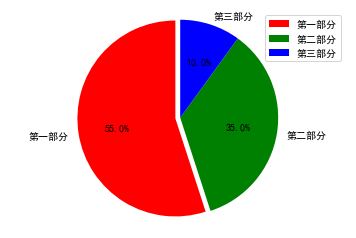展开全文• 利用 matplotlib 制作条形图 排列在工作表的列或行中的数据可以绘制到柱状图(条形图)中。 特点：绘制连离散的数据,能够一眼看出各个数据的大小,比较数据之间的差别。(统计/对比) 我们用一个例子来描述利用matplotlib...
• 采用ggplot2绘制折线图和条形图,并添加误差线.ggplot2只能处理 data.frame数据,每列作为一个变量,是一个指标.以ToothGrowth数据为例,进行处理tg ToothGrowth head(tg)## len supp dose ## 1 4.2 VC 0.5 ## 2 11.5 VC...

本文更新地址: http://blog.csdn.net/tanzuozhev/article/details/51106089

本文在 http://www.cookbook-r.com/Graphs/Plotting_means_and_error_bars_(ggplot2)/ 的基础上加入了自己的理解

采用ggplot2绘制折线图和条形图,并添加误差线.
ggplot2只能处理 data.frame数据,每列作为一个变量,是一个指标.

以ToothGrowth数据为例,进行处理
tg <- ToothGrowth
head(tg)
##    len supp dose
## 1  4.2   VC  0.5
## 2 11.5   VC  0.5
## 3  7.3   VC  0.5
## 4  5.8   VC  0.5
## 5  6.4   VC  0.5
## 6 10.0   VC  0.5
library(ggplot2)

数据预处理
采用summarySE()(函数定义在本文末尾)对数据进行预处理,计算数据的 标准误差(Standard Error). > 标准误差区别于标准差(Standard Deviation) . SE为 standard error of the mean 可以参考: http://blog.csdn.net/tanzuozhev/article/details/50830928
# summarySE 计算标准差和标准误差以及95%的置信区间.

tgc <- summarySE(tg, measurevar="len", groupvars=c("supp","dose"))
tgc
##   supp dose  N   len       sd        se       ci
## 1   OJ  0.5 10 13.23 4.459709 1.4102837 3.190283
## 2   OJ  1.0 10 22.70 3.910953 1.2367520 2.797727
## 3   OJ  2.0 10 26.06 2.655058 0.8396031 1.899314
## 4   VC  0.5 10  7.98 2.746634 0.8685620 1.964824
## 5   VC  1.0 10 16.77 2.515309 0.7954104 1.799343
## 6   VC  2.0 10 26.14 4.797731 1.5171757 3.432090

折线图
绘制带有误差线和95%置信区间线的折线图和点图
# 带有标准误差线的折线图
# Standard error of the mean
ggplot(tgc, aes(x=dose, y=len, colour=supp)) +
geom_errorbar(aes(ymin=len-se, ymax=len+se), width=.1) +
geom_line() +
geom_point()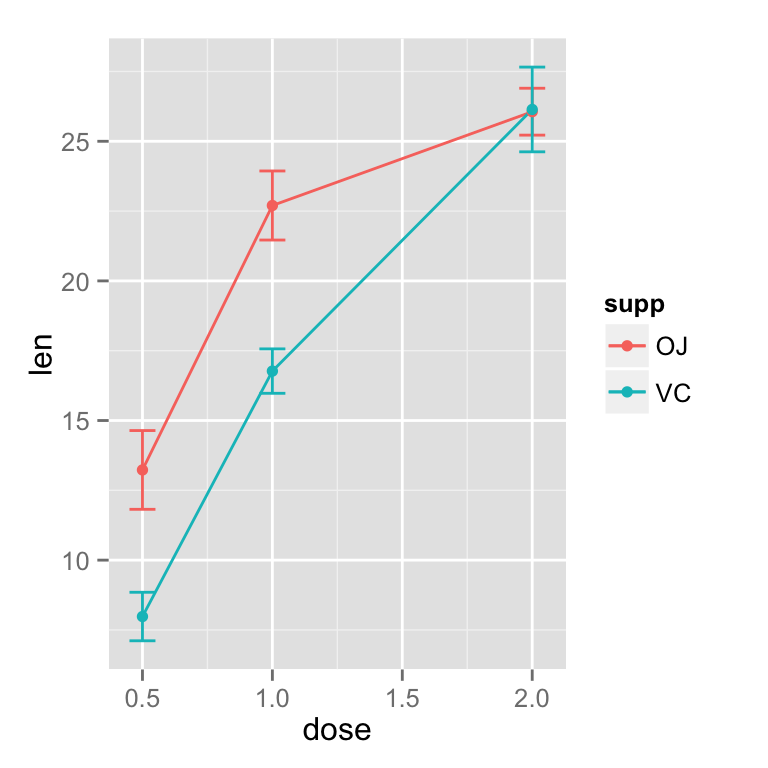# 对重叠的点,进行偏移处理(尽管这样可以将点分开便于观看,但是个人认为这并不科学)
pd <- position_dodge(0.1) # move them .05 to the left and right

ggplot(tgc, aes(x=dose, y=len, colour=supp)) +
geom_errorbar(aes(ymin=len-se, ymax=len+se), width=.1, position=pd) +
geom_line(position=pd) +
geom_point(position=pd)
## ymax not defined: adjusting position using y instead
## ymax not defined: adjusting position using y instead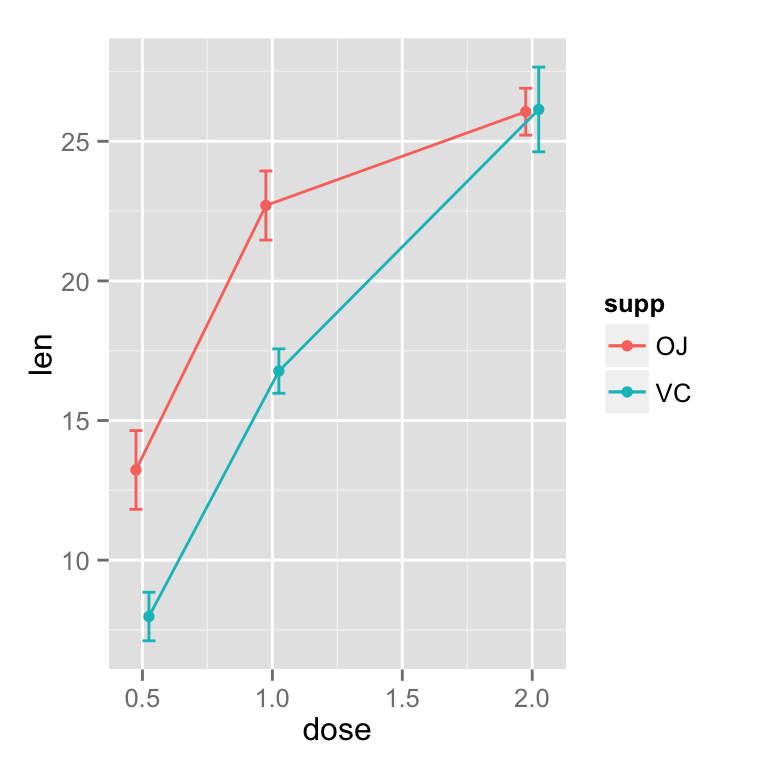# 绘制带有95%置信区间的折线图
ggplot(tgc, aes(x=dose, y=len, colour=supp)) +
geom_errorbar(aes(ymin=len-ci, ymax=len+ci), width=.1, position=pd) +
geom_line(position=pd) +
geom_point(position=pd)
## ymax not defined: adjusting position using y instead
## ymax not defined: adjusting position using y instead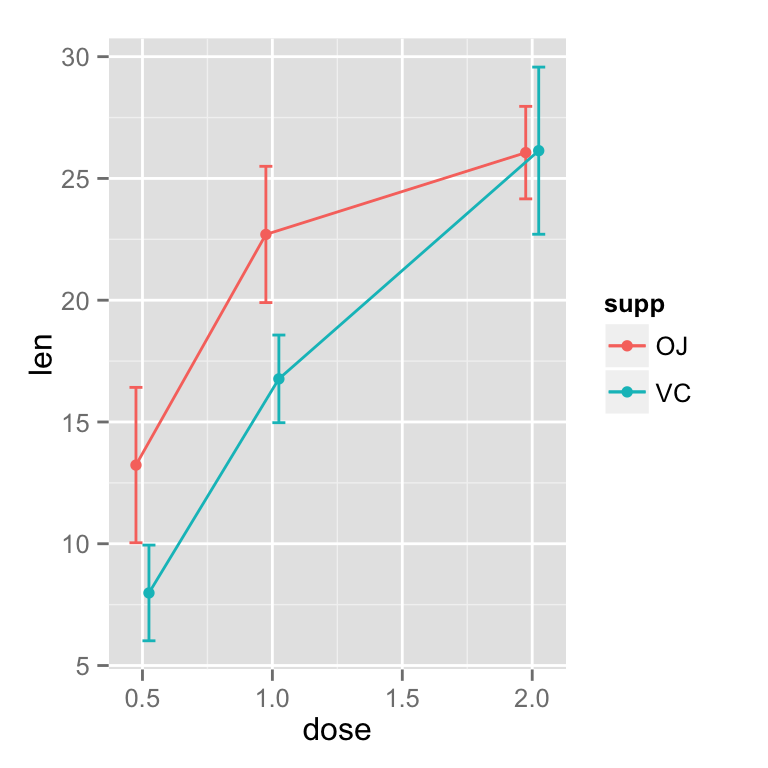# 设置误差线的颜色,特别注意如果没有 group=supp,这个重合的误差线将不会偏移.

ggplot(tgc, aes(x=dose, y=len, colour=supp, group=supp)) +
geom_errorbar(aes(ymin=len-ci, ymax=len+ci), colour="black", width=.1, position=pd) +
geom_line(position=pd) +
geom_point(position=pd, size=3)
## ymax not defined: adjusting position using y instead
## ymax not defined: adjusting position using y instead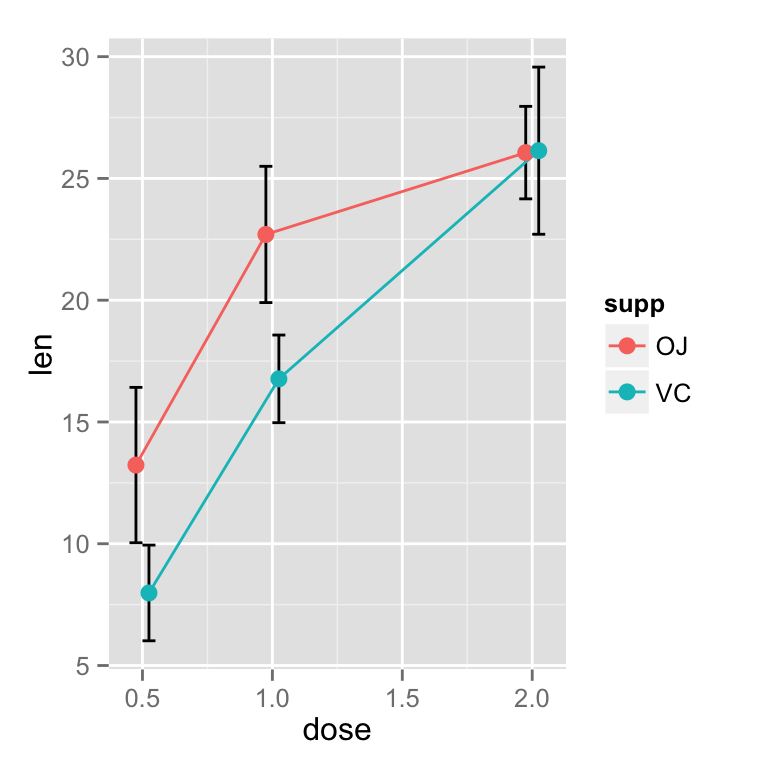下面是一个完整的带有标准误差线的图,geom_point 放在 geom_line之后,可以保证点被最后绘制,填充为白色.
ggplot(tgc, aes(x=dose, y=len, colour=supp, group=supp)) +
geom_errorbar(aes(ymin=len-se, ymax=len+se), colour="black", width=.1, position=pd) +
geom_line(position=pd) +
geom_point(position=pd, size=3, shape=21, fill="white") + # 21 is filled circle
xlab("Dose (mg)") +
ylab("Tooth length") +
scale_colour_hue(name="Supplement type",    # Legend label, use darker colors
breaks=c("OJ", "VC"),
labels=c("Orange juice", "Ascorbic acid"),
l=40) +                    # Use darker colors, lightness=40
ggtitle("The Effect of Vitamin C on\nTooth Growth in Guinea Pigs") +
expand_limits(y=0) +                        # Expand y range
scale_y_continuous(breaks=0:20*4) +         # Set tick every 4
theme_bw() +
theme(legend.justification=c(1,0),# 这一项很关键,如果没有这个参数,图例会偏移,读者可以试一试
legend.position=c(1,0))               # Position legend in bottom right
## ymax not defined: adjusting position using y instead
## ymax not defined: adjusting position using y instead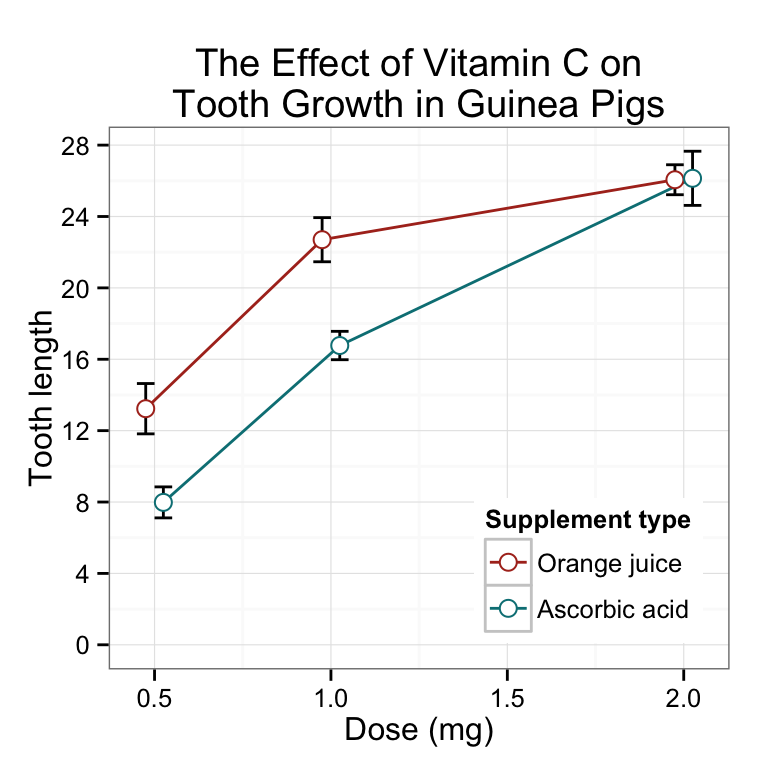条形图
绘制条形图与绘制折线图类似,但是必须要注意的是tgc$size必须被设置成 factor 类型,如果它是 数值型向量,那么将会出现错误. > 这是因为dose如果是 数值型向量将会作为连续型数据进行处理,而 因子型 变量被作为离散型数据进行处理. # 转换为因子类型 tgc2 <- tgc tgc2$dose <- factor(tgc2$dose) # Error bars represent standard error of the mean ggplot(tgc2, aes(x=dose, y=len, fill=supp)) + geom_bar(position=position_dodge(), stat="identity") + geom_errorbar(aes(ymin=len-se, ymax=len+se), width=.2, # 设置误差线的宽度 position=position_dodge(.9))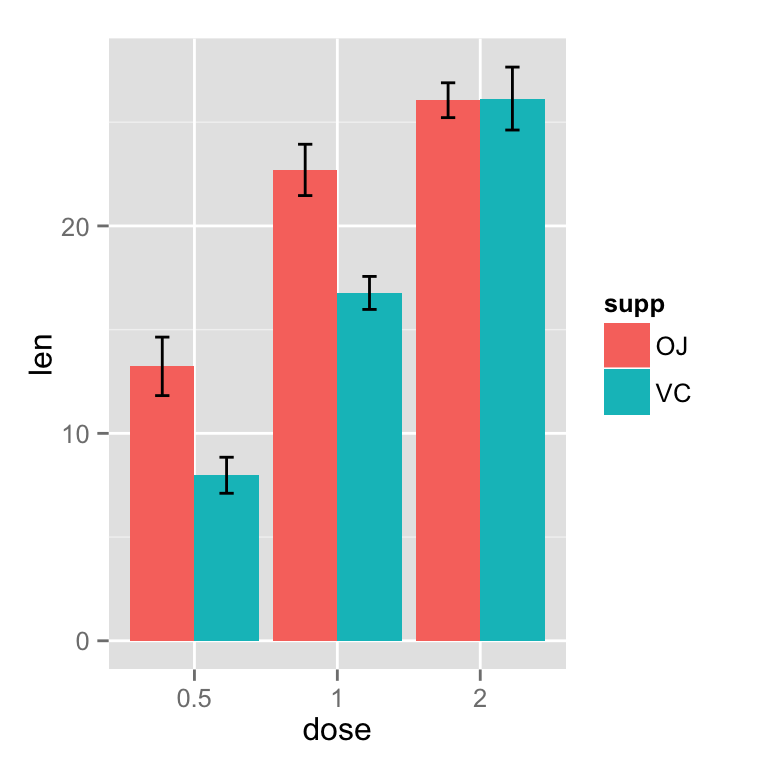# 使用95%置信区间 ggplot(tgc2, aes(x=dose, y=len, fill=supp)) + geom_bar(position=position_dodge(), stat="identity") + geom_errorbar(aes(ymin=len-ci, ymax=len+ci), width=.2, # Width of the error bars position=position_dodge(.9))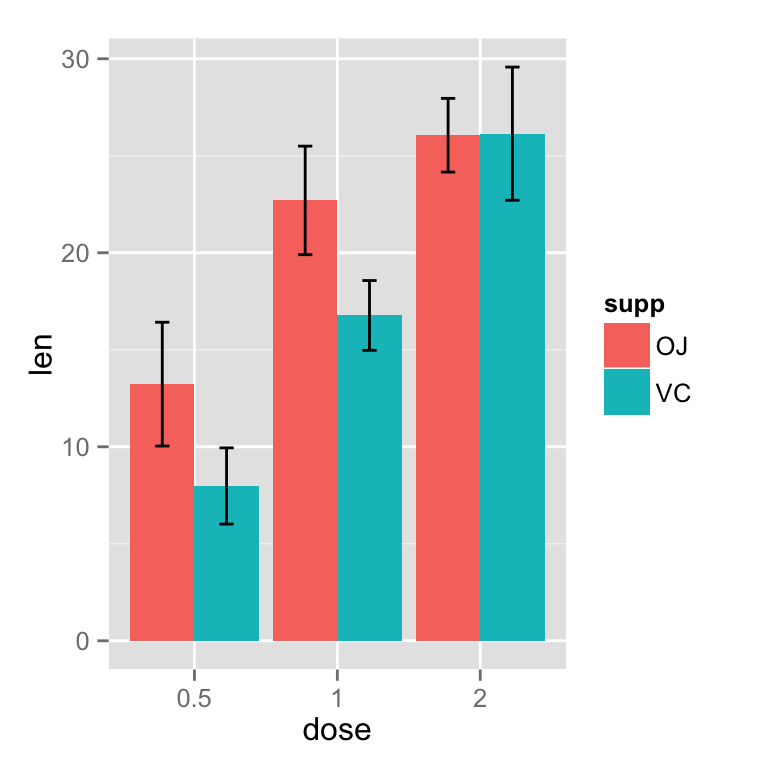完整的条形图 ggplot(tgc2, aes(x=dose, y=len, fill=supp)) + geom_bar(position=position_dodge(), stat="identity", colour="black", # Use black outlines, size=.3) + # Thinner lines geom_errorbar(aes(ymin=len-se, ymax=len+se), size=.3, # Thinner lines width=.2, position=position_dodge(.9)) + xlab("Dose (mg)") + ylab("Tooth length") + scale_fill_hue(name="Supplement type", # Legend label, use darker colors breaks=c("OJ", "VC"), labels=c("Orange juice", "Ascorbic acid")) + ggtitle("The Effect of Vitamin C on\nTooth Growth in Guinea Pigs") + scale_y_continuous(breaks=0:20*4) + theme_bw()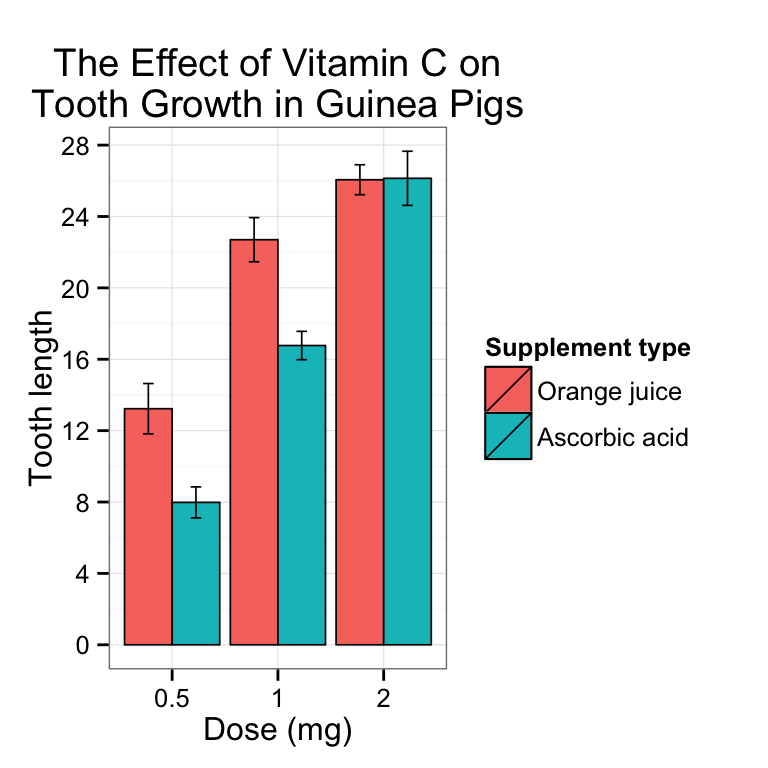## Gives count, mean, standard deviation, standard error of the mean, and confidence interval (default 95%). ## data: a data frame. ## measurevar: the name of a column that contains the variable to be summariezed ## groupvars: a vector containing names of columns that contain grouping variables ## na.rm: a boolean that indicates whether to ignore NA's ## conf.interval: the percent range of the confidence interval (default is 95%) summarySE <- function(data=NULL, measurevar, groupvars=NULL, na.rm=FALSE, conf.interval=.95, .drop=TRUE) { library(plyr) # 计算长度 length2 <- function (x, na.rm=FALSE) { if (na.rm) sum(!is.na(x)) else length(x) } # 以 groupvars 为组,计算每组的长度,均值,以及标准差 # ddply 就是 dplyr 中的 group_by + summarise datac <- ddply(data, groupvars, .drop=.drop, .fun = function(xx, col) { c(N = length2(xx[[col]], na.rm=na.rm), mean = mean (xx[[col]], na.rm=na.rm), sd = sd (xx[[col]], na.rm=na.rm) ) }, measurevar ) # 重命名 datac <- plyr::rename(datac, c("mean" = measurevar)) # 计算标准偏差 datac$se <- datac$sd / sqrt(datac$N)  # Calculate standard error of the mean

# Confidence interval multiplier for standard error
# Calculate t-statistic for confidence interval:
# e.g., if conf.interval is .95, use .975 (above/below), and use df=N-1
# 计算置信区间
ciMult <- qt(conf.interval/2 + .5, datac$N-1) datac$ci <- datac\$se * ciMult

return(datac)
}

展开全文ggplot2 R语言 cookbook
• 遵循以下列出的要求，使用d3.js制作的条形图： X轴是-z。 Y轴为-10到90（条形图可以位于x轴下方）。 X轴没有任何刻度。 在该示例中，没有下拉菜单，而是有一个接受数字的输入框。 范围是（0到10）。 默认情况下，...JavaScript
• 此函数为 MxN 矩阵中的每个单独的条形创建一个带有任意颜色的 3D 条形图。 条形高度 = 矩阵的元素条形颜色 = (1) 用户指定的颜色图或 (2) RGB 颜色向量的 MxNx3 数组。 如果您想进行进一步更改，它会返回单个条形图...matlab
• 这是一张微立体风格的，饼状图条形图搭配PPT图表。左侧是微立体四数据项PPT饼状图，右侧搭配扁平化条形图对比展示。.PPTX格式；
• 16、17、18_使用gridspec定义多子图,条形图（Bar plots）,分组条形图,堆叠条形图(Stacked bar chart),饼图（Pie plots）,甜甜圈图,嵌套饼图.pdf
• 这是两张彩色4数据线对比的，实用PPT条形图，.PPTX格式；
• 本文实例为大家分享了python绘制水平条形图的具体代码，供大家参考，具体内容如下 水平条形图与绘制柱状图类似，大家可以先看看我之前写的博客，如何绘制柱状图 水平条形图需要在Bar函数中设置orientation= ‘h’ ...
• 增长幅度统计PPT条形图素材下载，关键词：柱状图，条状图，条形图，统计数据，PPT图表，幻灯片素材下载，PPTX格式； 增长幅度统计PPT条形图素材下载，关键词：柱状图，条状图，条形图，统计数据，PPT图表，幻灯片......